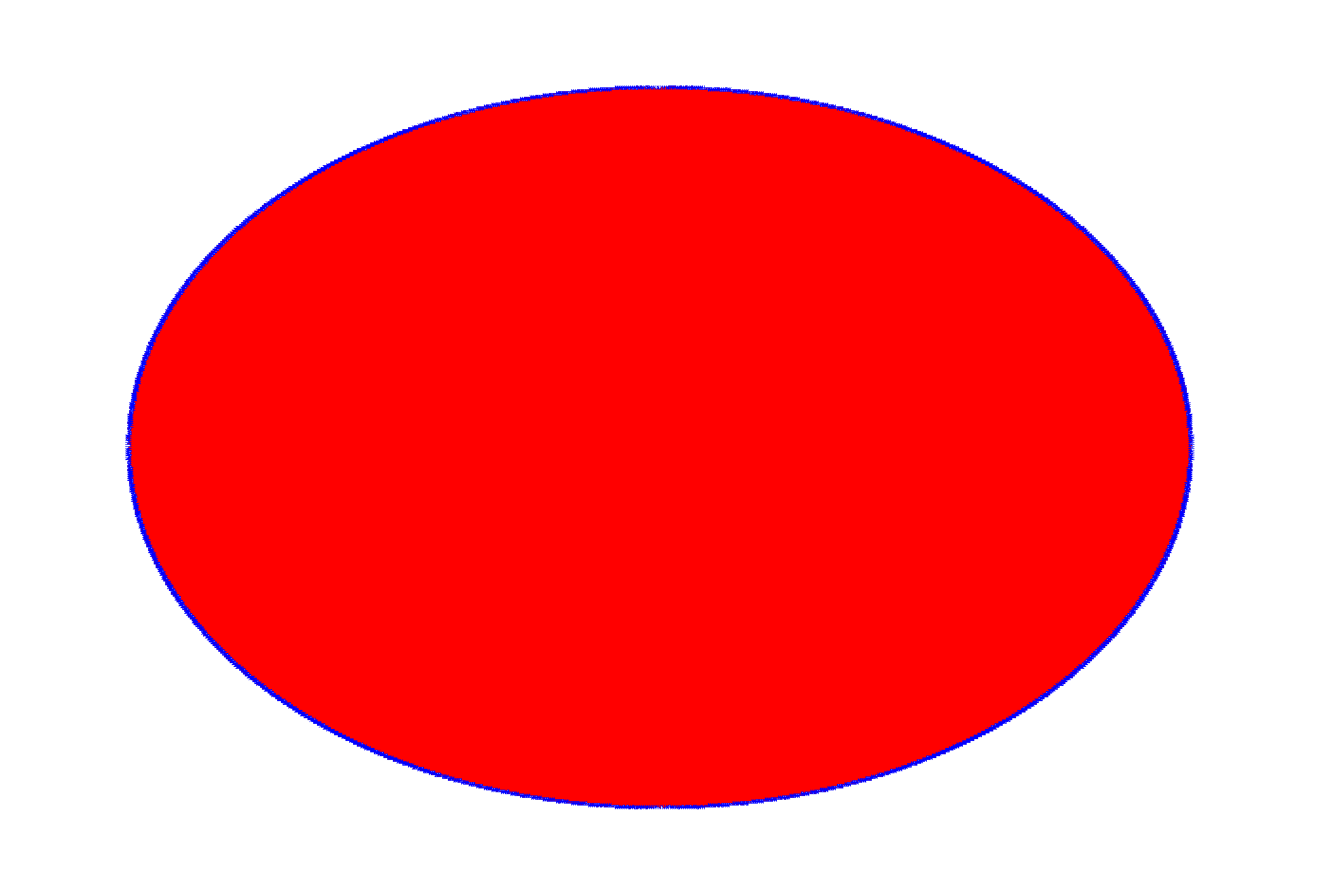ROOT   Reference Guide
Searching...
No Matches
polytest1.C File Reference

## Detailed DescriptionThis macro is testing the "compacting" algorithm in TPadPainter.

It reduces the number of polygon's vertices using actual pixel coordinates.It's not really useful, but just to test that the resulting polygon is still reasonable. Initial number of points is 1000000, after "compression" it's 523904 (with default canvas size, before you tried to resize it) - so almost half of vertices were removed but you can still see the reasonable shape. If you resize a canvas to a smaller size, the number of vertices after compression can be something like 5000 and even less. It's easy to 'fool' this algorithm though in this particular case (ellipse is a kind of fringe case, you can easily have a sequence of almost unique vertices (at a pixel level).

//Includes for ACLiC.
#include <cassert>
#include <vector>
#include "TRandom.h"
#include "TCanvas.h"
#include "TError.h"
#include "Rtypes.h"
#include "TNamed.h"
#include "TMath.h"
class PolyTest1 : public TNamed, public TAttLine, public TAttFill {
public:
PolyTest1(unsigned nVertices);
void Paint(const Option_t *notUsed);
void Reset(unsigned nVertices);
private:
enum {
kNPointsDefault = 10000//minimal number of points.
};
std::vector<Double_t> fXs;
std::vector<Double_t> fYs;
};
//_____________________________________________________________
PolyTest1::PolyTest1(unsigned nVertices)
: TNamed("polygon_compression_test1", "polygon_compression_test1")
{
Reset(nVertices);
}
//_____________________________________________________________
void PolyTest1::Reset(unsigned nVertices)
{
//Some canvas must already exist by this point.
//We need a gRandom to exist.
assert(gRandom != 0 && "Reset, gRandom is null");
if (nVertices < kNPointsDefault) {
Warning("Reset", "resetting nVertices parameter to %u", unsigned(kNPointsDefault));
nVertices = kNPointsDefault;
}
fXs.resize(nVertices);
fYs.resize(nVertices);
Double_t xMin = 0., xMax = 0., yMin = 0., yMax = 0.;
assert(xMax - xMin > 0 && yMax - yMin > 0 && "Reset, invalid canvas' ranges");
const Double_t xCentre = xMin + 0.5 * (xMax - xMin);
const Double_t yCentre = yMin + 0.5 * (yMax - yMin);
const Double_t r = TMath::Min(xMax - xMin, yMax - yMin) * 0.8 / 2;
const Double_t angle = TMath::TwoPi() / (nVertices - 1);
for (unsigned i = 0; i < nVertices - 1; ++i) {
const Double_t currR = r + gRandom->Rndm() * r * 0.01;
fXs[i] = xCentre + currR * TMath::Cos(angle * i);
fYs[i] = yCentre + currR * TMath::Sin(angle * i);
}
fXs[nVertices - 1] = fXs;
fYs[nVertices - 1] = fYs;
}
//_____________________________________________________________
void PolyTest1::Paint(const Option_t * /*notUsed*/)
{
}
void polytest1()
{
TCanvas * const cnv = new TCanvas;
cnv->cd();
PolyTest1 * polygon = new PolyTest1(1000000);
polygon->SetLineColor(kBlue);
polygon->SetFillColor(kRed);
polygon->SetLineWidth(1);
polygon->Draw();//Attach a polygon to a canvas.
}
ROOT::R::TRInterface & r
Definition Object.C:4
int Int_t
Definition RtypesCore.h:45
double Double_t
Definition RtypesCore.h:59
const char Option_t
Definition RtypesCore.h:66
@ kRed
Definition Rtypes.h:66
@ kBlue
Definition Rtypes.h:66
void Warning(const char *location, const char *msgfmt,...)
Use this function in warning situations.
Definition TError.cxx:231
R__EXTERN TRandom * gRandom
Definition TRandom.h:62
Fill Area Attributes class.
Definition TAttFill.h:19
virtual void Modify()
Change current fill area attributes if necessary.
Definition TAttFill.cxx:211
Line Attributes class.
Definition TAttLine.h:18
virtual void Modify()
Change current line attributes if necessary.
Definition TAttLine.cxx:242
The Canvas class.
Definition TCanvas.h:23
Definition TCanvas.cxx:708
The TNamed class is the base class for all named ROOT classes.
Definition TNamed.h:29
virtual void Paint(Option_t *option="")
This method must be overridden if a class wants to paint itself.
Definition TObject.cxx:521
virtual Double_t Rndm()
Machine independent random number generator.
Definition TRandom.cxx:552
Short_t Min(Short_t a, Short_t b)
Definition TMathBase.h:180
Double_t Cos(Double_t)
Definition TMath.h:643
Double_t Sin(Double_t)
Definition TMath.h:639
constexpr Double_t TwoPi()
Definition TMath.h:44

Definition in file polytest1.C.